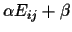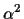Next: Example Up: Deriving the Optimal Scoring Previous: Variance of the estimator

## Degrees of Freedom

Because our model of evolution is symmetrical, i.e. it does not distinguish a particular sequence, E must be symmetric. It is also easy to see that if we replace Eij bythen F(d) does not change (both numerator and denominator are multiplied by). Before we do any minimization we have to eliminate these two degrees of freedom. Most of the time this can be done by arbitrarily setting two values of E, e.g. E11 = -1 and E12 = 1.

Chantal Korostensky
1999-07-14